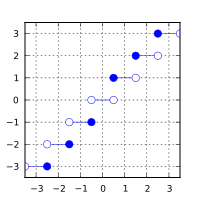/C

# round, roundf, roundl, lround, lroundf, lroundl, llround, llroundf, llroundl

Defined in header `<math.h>`
`float       roundf( float arg );`
(1) (since C99)
`double      round( double arg );`
(2) (since C99)
`long double roundl( long double arg );`
(3) (since C99)
Defined in header `<tgmath.h>`
`#define round( arg )`
(4) (since C99)
Defined in header `<math.h>`
`long      lroundf( float arg );`
(5) (since C99)
`long      lround( double arg );`
(6) (since C99)
`long      lroundl( long double arg );`
(7) (since C99)
Defined in header `<tgmath.h>`
`#define lround( arg )`
(8) (since C99)
Defined in header `<math.h>`
`long long llroundf( float arg );`
(9) (since C99)
`long long llround( double arg );`
(10) (since C99)
`long long llroundl( long double arg );`
(11) (since C99)
Defined in header `<tgmath.h>`
`#define llround( arg )`
(12) (since C99)
1-3) Computes the nearest integer value to `arg` (in floating-point format), rounding halfway cases away from zero, regardless of the current rounding mode.
5-7, 9-11) Computes the nearest integer value to `arg` (in integer format), rounding halfway cases away from zero, regardless of the current rounding mode.
4,8,12) Type-generic macros: If `arg` has type `long double`, `roundl`, `lroundl`, `llroundl` is called. Otherwise, if `arg` has integer type or the type `double`, `round`, `lround`, `llround` is called. Otherwise, `roundf`, `lroundf`, `llroundf` is called, respectively.

### Parameters

 arg - floating point value

### Return value

If no errors occur, the nearest integer value to `arg`, rounding halfway cases away from zero, is returned.

Return valueArgument

If a domain error occurs, an implementation-defined value is returned.

### Error handling

Errors are reported as specified in math_errhandling.

If the result of `lround` or `llround` is outside the range representable by the return type, a domain error or a range error may occur.

If the implementation supports IEEE floating-point arithmetic (IEC 60559), For the `round`, `roundf`, and `roundl` function:

• The current rounding mode has no effect.
• If `arg` is ±∞, it is returned, unmodified
• If `arg` is ±0, it is returned, unmodified
• If `arg` is NaN, NaN is returned
For `lround` and `llround` families of functions:
• `FE_INEXACT` is never raised
• The current rounding mode has no effect.
• If `arg` is ±∞, `FE_INVALID` is raised and an implementation-defined value is returned
• If the result of the rounding is outside the range of the return type, `FE_INVALID` is raised and an implementation-defined value is returned
• If `arg` is NaN, `FE_INVALID` is raised and an implementation-defined value is returned

`FE_INEXACT` may be (but isn't required to be) raised by `round` when rounding a non-integer finite value.

The largest representable floating-point values are exact integers in all standard floating-point formats, so `round` never overflows on its own; however the result may overflow any integer type (including `intmax_t`), when stored in an integer variable.

POSIX specifies that all cases where `lround` or `llround` raise `FE_INEXACT` are domain errors.

The `double` version of `round` behaves as if implemented as follows:

```#include <math.h>
#include <fenv.h>
#pragma STDC FENV_ACCESS ON
double round(double x)
{
fenv_t save_env;
feholdexcept(&save_env);
double result = rint(x);
if (fetestexcept(FE_INEXACT)) {
fesetround(FE_TOWARDZERO);
result = rint(copysign(0.5 + fabs(x), x));
}
feupdateenv(&save_env);
return result;
}```

### Example

```#include <stdio.h>
#include <math.h>
#include <fenv.h>
#include <limits.h>

#pragma STDC FENV_ACCESS ON

int main(void)
{
// round
printf("round(+2.3) = %+.1f  ", round(2.3));
printf("round(+2.5) = %+.1f  ", round(2.5));
printf("round(+2.7) = %+.1f\n", round(2.7));
printf("round(-2.3) = %+.1f  ", round(-2.3));
printf("round(-2.5) = %+.1f  ", round(-2.5));
printf("round(-2.7) = %+.1f\n", round(-2.7));

printf("round(-0.0) = %+.1f\n", round(-0.0));
printf("round(-Inf) = %+f\n",   round(-INFINITY));

// lround
printf("lround(+2.3) = %ld  ", lround(2.3));
printf("lround(+2.5) = %ld  ", lround(2.5));
printf("lround(+2.7) = %ld\n", lround(2.7));
printf("lround(-2.3) = %ld  ", lround(-2.3));
printf("lround(-2.5) = %ld  ", lround(-2.5));
printf("lround(-2.7) = %ld\n", lround(-2.7));

printf("lround(-0.0) = %ld\n", lround(-0.0));
printf("lround(-Inf) = %ld\n", lround(-INFINITY)); // FE_INVALID raised

// error handling
feclearexcept(FE_ALL_EXCEPT);
printf("lround(LONG_MAX+1.5) = %ld\n", lround(LONG_MAX+1.5));
if(fetestexcept(FE_INVALID)) puts("    FE_INVALID was raised");
}```

Possible output:

```round(+2.3) = +2.0  round(+2.5) = +3.0  round(+2.7) = +3.0
round(-2.3) = -2.0  round(-2.5) = -3.0  round(-2.7) = -3.0
round(-0.0) = -0.0
round(-Inf) = -inf
lround(+2.3) = 2  lround(+2.5) = 3  lround(+2.7) = 3
lround(-2.3) = -2  lround(-2.5) = -3  lround(-2.7) = -3
lround(-0.0) = 0
lround(-Inf) = -9223372036854775808
lround(LONG_MAX+1.5) = -9223372036854775808
FE_INVALID was raised```
• C11 standard (ISO/IEC 9899:2011):
• 7.12.9.6 The round functions (p: 253)
• 7.12.9.7 The lround and llround functions (p: 253)
• 7.25 Type-generic math <tgmath.h> (p: 373-375)
• F.10.6.6 The round functions (p: 527)
• F.10.6.7 The lround and llround functions (p: 528)
• C99 standard (ISO/IEC 9899:1999):
• 7.12.9.6 The round functions (p: 233)
• 7.12.9.7 The lround and llround functions (p: 234)
• 7.22 Type-generic math <tgmath.h> (p: 335-337)
• F.9.6.6 The round functions (p: 464)
• F.9.6.7 The lround and llround functions (p: 464)